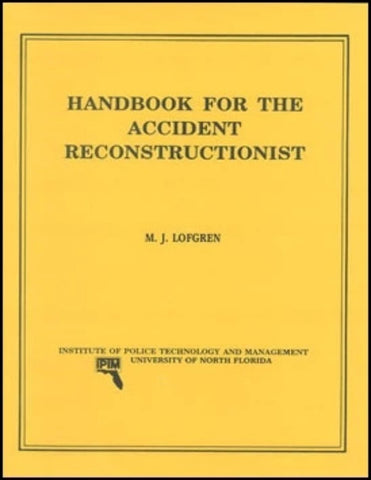# Handbook for the Accident Reconstructionist

• \$ 5.00

### Handbook for the Accident Reconstructionist by M.J. Lofgren

Mr. Lofgren consolidates many of the scientific techniques used in crash reconstruction and puts them together for you in one reference manual. He simplifies formulas, where possible, and explains the principles behind each. He also includes examples and works step-by-step through each problem.

If your math skills are a bit rusty, Mr. Lofgren includes a 104-page appendix devoted entirely to a course in refresher mathematics. From there, he delves into the equations used for determining speed, kinetic energy, weight shift and other aspects of crash reconstruction. As a bonus, he’ll tell you which formulas will come under scrutiny in court and which have already been tested.

Handbook for the Accident Reconstructionist includes the following chapters:

• Geometric Construction
• Minimum Speed from Skidmarks
• Fall Speed
• Derivation of the Vault Formula
• Derivation of the Critical Speed Formula
• Kinetic Energy Equation
• Derivation of the Radius Equation